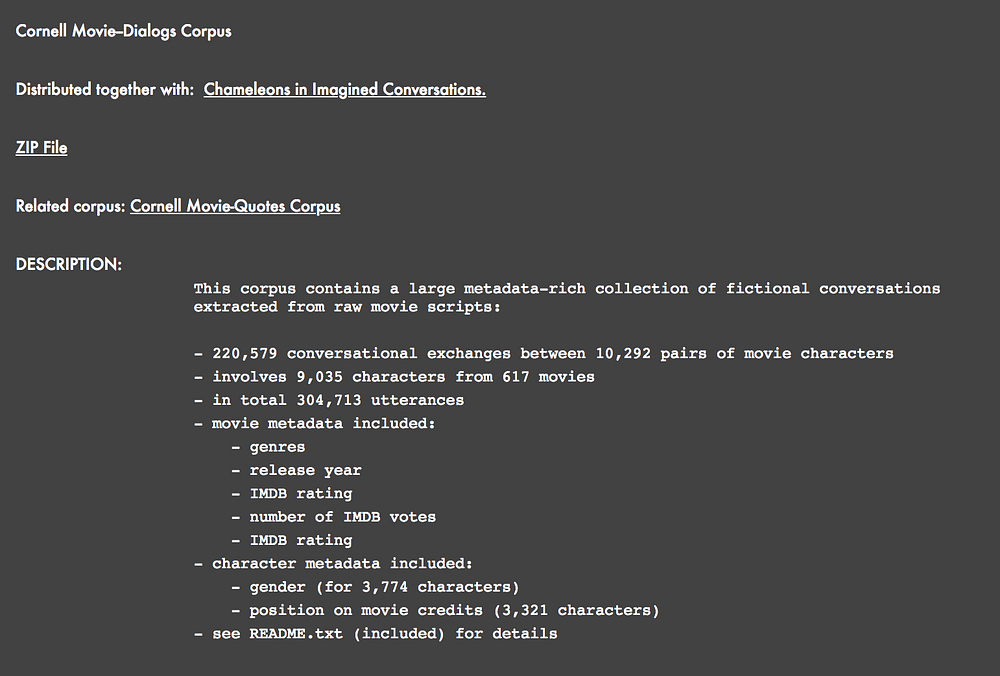# The Art of Building a Chatbot

ModelingNLP/Text Analyticsposted by Alexander Osipenko September 17, 2018

With the development of deep learning and NLP chatbots become more and more popular. The hype for chatbots is already high...With the development of deep learning and NLP chatbots become more and more popular. The hype for chatbots is already high and it will be increasing for the next several years.

“By 2020, over 50% of medium to large enterprises will have deployed product chatbots” — Van Baker, research vice president at Gartner

With Google’s release of the Sequence to Sequence Learning with Neural Networks paper in 2014 and rapid development of open source tools such us Tensor Flow, chatbots become easier to build.

#### 1. First thing first — gathering the data

There are many different open-source datasets available. Probably the most popular is Cornell Movie — Dialogs Corpus and I used this dataset too. But for example, a much bigger dataset based on Reddit comments can be found here.#### 2. Preparing your data

One of the most challenging thing in NLP for me is data preparation. In order to be recognizable for the Neural Network words needs to be represented as vectors. There are different ways to do that, for example, Gensim, but I used Keras implementation of TensorFlow’s embedding.

I loaded data as a list of sentences.

Then I extracted all unique words from the text and assign a unique integer number for each word.

So, as a result, I’ve got a list of lists with encoded words, where each word represents a number. For example, vector `[42, 14, 35, 18]` represents text: “They do not!” and `` represents “Wow”

So far so good! Now we face a problem that every line of text (vector) has different length, so Neural Network can’t be trained by this. A common solution is to replace each vector with a dummy matrix, filled with zeros, so instead of having vectors with different lengths, we will have matrixes with the same dimensionality, and then incorporate each vector into this matrixes.

#### 3. Compiling your model

For the model, I used Neural network with seq2seq architecture (see picture from cover). This model contains two parts Encoder and Decoder each of them is a set of LSTM cells. Encoder and decoder can share weights or use a different set of parameters.

Define encoder:

```encoder_inputs = Input(shape=(None,), name='encoder_inputs')
encoder_embedding = Embedding(input_dim=num_encoder_tokens, output_dim=HIDDEN_UNITS,input_length=encoder_max_seq_length, name='encoder_embedding')
encoder_lstm = LSTM(units=HIDDEN_UNITS, return_state=True, name='encoder_lstm')
encoder_outputs, encoder_state_h, encoder_state_c = encoder_lstm(encoder_embedding(encoder_inputs))
encoder_states = [encoder_state_h, encoder_state_c]```

Define decoder:

```decoder_inputs = Input(shape=(None, num_decoder_tokens), name='decoder_inputs')
decoder_lstm = LSTM(units=HIDDEN_UNITS, return_state=True, return_sequences=True, name='decoder_lstm')
decoder_outputs, decoder_state_h, decoder_state_c = decoder_lstm(decoder_inputs,                                        initial_state=encoder_states)
decoder_dense = Dense(units=num_decoder_tokens, activation='softmax', name='decoder_dense')
decoder_outputs = decoder_dense(decoder_outputs)```

Compile my model:

`model = Model([encoder_inputs, decoder_inputs], decoder_outputs)`
`model.compile(loss='categorical_crossentropy', optimizer='rmsprop')`
```json = model.to_json()
open('model/word-architecture.json', 'w').write(json)```
`X_train, X_test, y_train, y_test = train_test_split(encoder_input_data, target_texts, test_size=0.2, random_state=42)`
```train_gen = generate_batch(X_train, y_train)
test_gen = generate_batch(X_test, y_test)```
```train_num_batches = len(X_train) // BATCH_SIZE
test_num_batches = len(X_test) // BATCH_SIZE```
```checkpoint = ModelCheckpoint(filepath=WEIGHT_FILE_PATH, save_best_only=True)
tbCallBack = TensorBoard(log_dir=TENSORBOARD, histogram_freq=0, write_graph=True, write_images=True)```

#### 4. Train my model

I used GPU 1080Ti but it still took a while for 100 epochs.

```model.fit_generator(generator=train_gen,
steps_per_epoch=train_num_batches,
epochs=NUM_EPOCHS,
verbose=1,
validation_data=test_gen,
validation_steps=test_num_batches,
callbacks=[checkpoint, tbCallBack ])```
`model.save_weights(WEIGHT_FILE_PATH)`

#### 5. Testing my chatbot!

So the model is trained! I made a class wrapper for it, so it will be more convenient to use. Method `reply` taking a text as input, transform it into vector, in the same way, I did in the training step and then call `predict` on the trained model.

Let’s try Turing Test!

Shame, but looks like my bot already did not pass this test.

Let’s try asking the Ultimate question

Wow! Amazing!

But to be honest I hardcoded that one as an easter egg…

That’s it! I think it confirms that my bot suffers from depression.

### What can be done next?

Code for this chatbot can be found here: https://github.com/subpath/ChatBot

1. If you have free time and computational power you can train the model on a bigger dataset like the one from Reddit
2. You can wrap it in some service maybe web service based on a flask or telegram bot

I will try to improve the quality of this bot by tweaking of hyperparameters. And probably will make telegram bot out of it in future.

### References:

Original article here.## Alexander Osipenko

Data Scientist with many years of experience in Computational Chemistry and Time-Series analysis. Passionate about Deep Learning and Data Science. I believe that Fourth Industrial Revolution is happening right now with rise of Big Data and Decentralised systems I'm proud to be the Lead of ML team at Cindicator, the company that stands on the edge of global changes. Links: https://medium.com/@subpath , https://twitter.com/subpath email: osipenko@cindicator.com

1